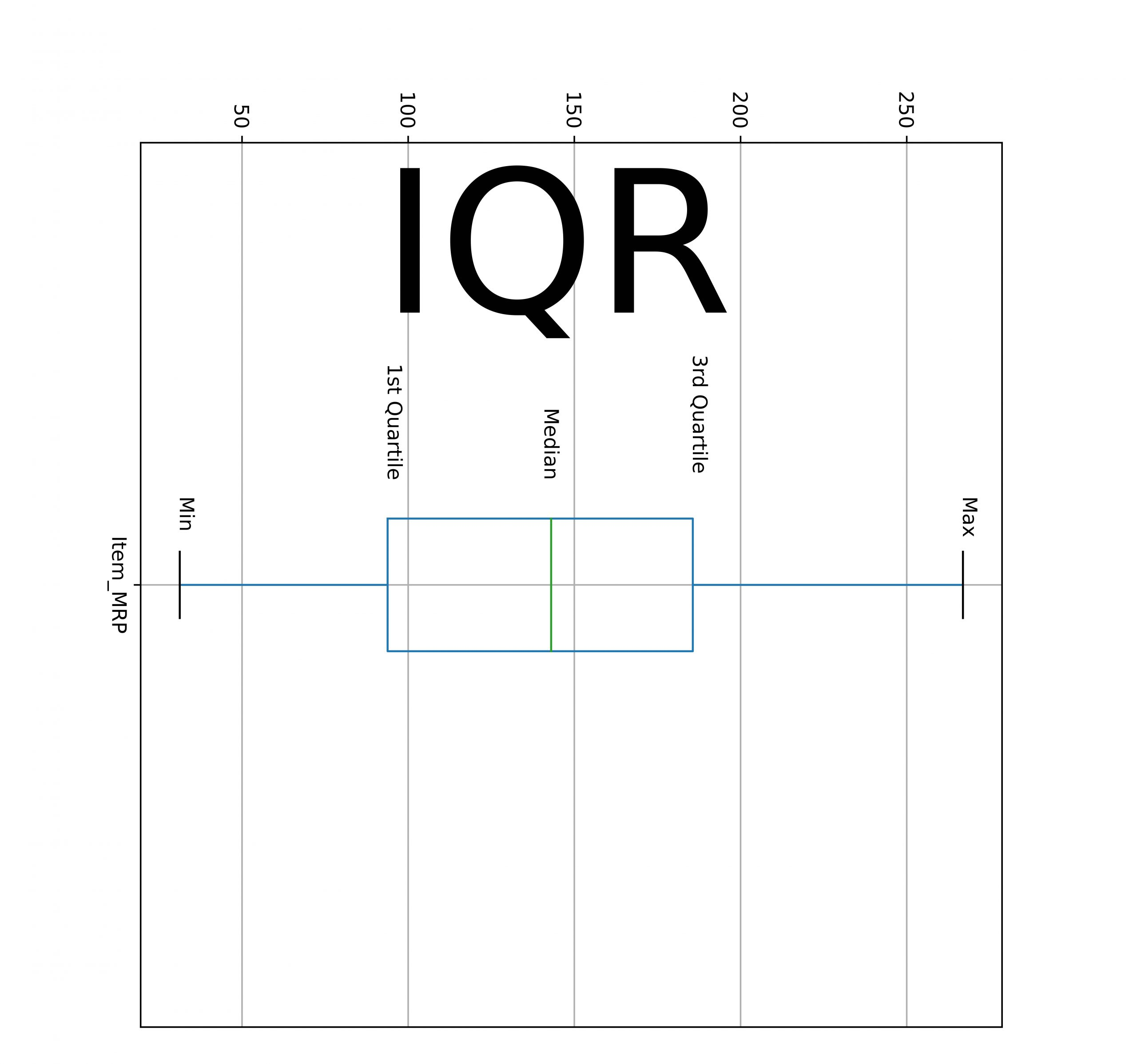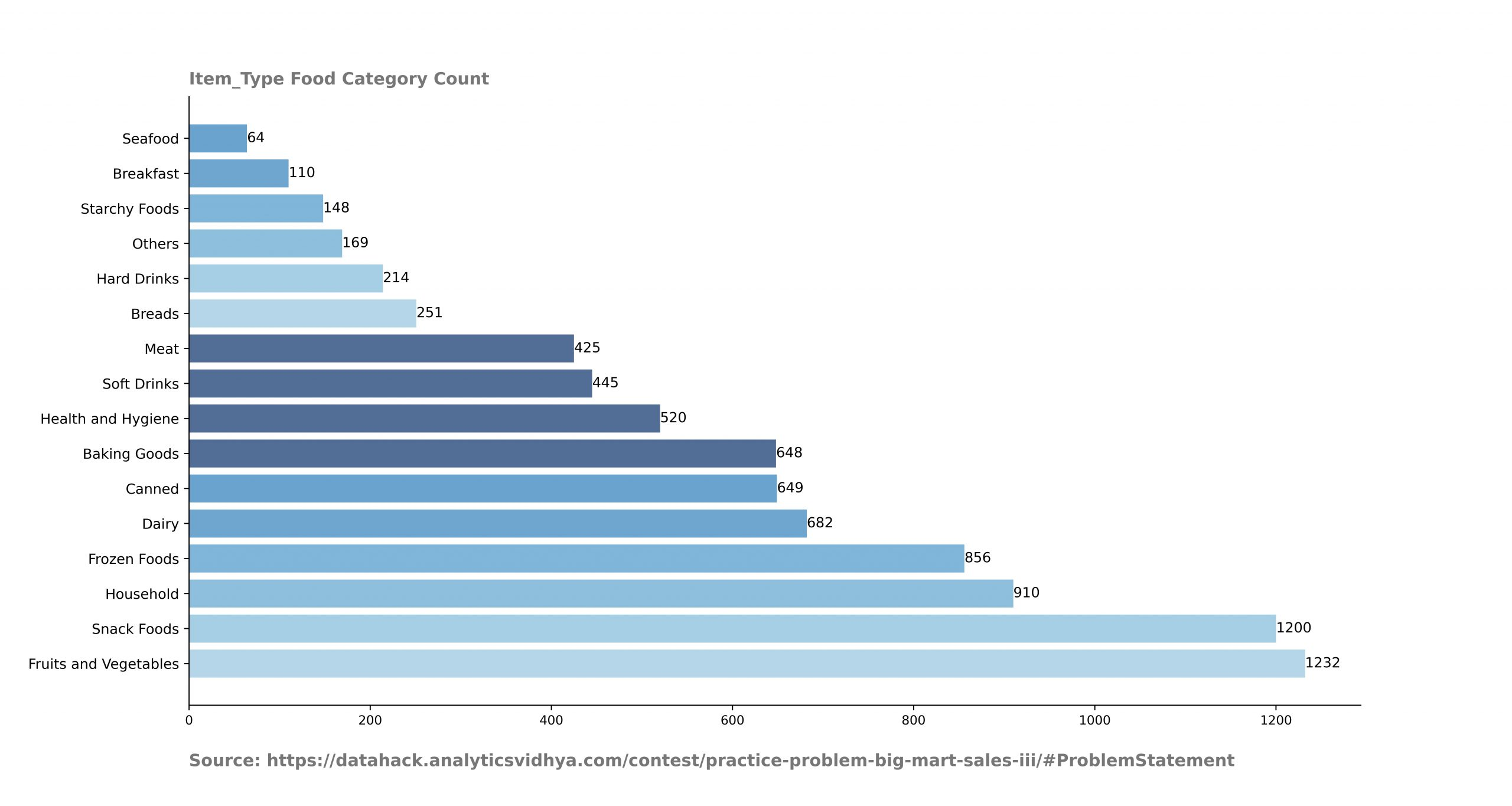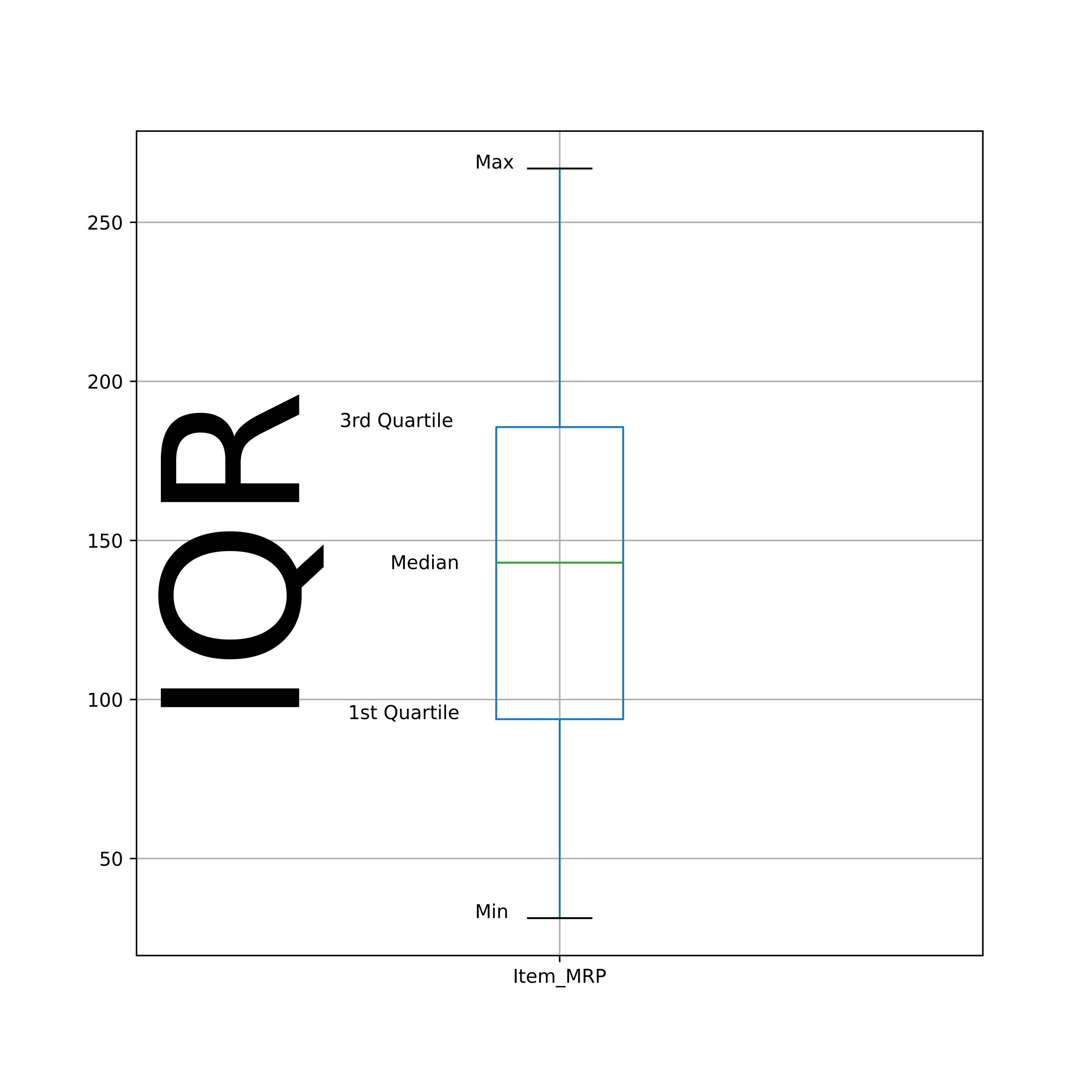Manmohan24nov — Updated On August 26th, 2022

This article was published as a part of the Data Science Blogathon.

## IntroductionThe first step in a data science project is to summarize, describe, and visualize the data. Try to know about different aspects of data and its attributes. Best models are created by those who understand their data.

Explore the features of data and its attributes using Descriptive Statistics. Insights and numerical Summary you get from Descriptive Statistics help you to better understand or be in a position to handle the data more efficiently for machine learning tasks.

Descriptive Statistics is the default process in Data analysis. Exploratory Data Analysis (EDA) is not complete without a Descriptive Statistic analysis.

So, in this article, I will explain the attributes of the dataset using Descriptive Statistics. It is divided into two parts: Measure of Central Data points and Measure of Dispersion. Before we start with our analysis, we need to complete the data collection and cleaning process.

## Data Collection and Data cleaning

We will collect data from here. I will only use test data for analysis. You can combine both test and train data for analysis. Here is a code for the data cleaning process of train data.

Python Code:

Take Away from the code

•  Column Item_Weight and Outlet_Size have null values. These are the options:
1.  remove the rows containing null values
2.  remove the columns containing null values
3.  or replace the null values.
• The first 2 options are feasible when data has rows in million or count of values is small. So, I will choose the 3rd option to solve the null value issue.
•  First, find the Item_Identifier and their corresponding Item_Weight. Then replace the missing/null in Item_Weight with the known Item_weight of the respective item_identifier.
•  As we know, the visibility of items in a store can be near zero but not zero. So, we consider 0 as a null value and follow the above step for Item_Visibility.
•  Outlet_Size does not hold much importance in our analysis and model prediction. So, I am dropping this column.
•  Replace LF and reg in Item_fat_content column with Low Fat and Regular Fat.
•  Calculate the age of stores and save these values in column Outlet_years and drop the Outlet_Establishment_year column.

## The Measure of Central Datapoint

Finding the center of numerical and categorical data using mean, median and mode is known as Measure of Central Datapoint. The calculation of central values of column data by mean, median, and mode are different from each other.

Okay then, let’s calculate the mean, median, count, and mode of the dataset attributes using python.

1. Count
The count does not help directly in finding the center of dataset attributes. But it is used in mean, median, and mode calculation. We calculate the total count in each category of the categorical variables. It also calculates the total count of numerical column data.#### Take away from the code.

•   Loop through the categorical columns to plot the category and its count.

#### Analysis of the output.

•  These counts help you to find out whether data is balanced or not. From this graph, I can say that rows of Fruits and Vegetable category are way more than the seafood category.
•  We can also assume that sales under the Fruits and vegetable category are way more than the seafood category.

2. #### Mean

The Sum of values present in the column divided by total rows of that column is known as mean. It is also known as average.

Use train.mean() to calculate the mean value of numerical columns of the train dataset.

Here is a code for categorical columns of the train dataset.

`print(train[['Item_Outlet_Sales','Outlet_Type']].groupby(['Outlet_Type']).agg({'Item_Outlet_Sales':'mean'}))`

Analysis of the output

• The average outlet age is 15 years.
• The average outlet sales are 2100.
• The supermarket type 3 category of Outlet_Type has way more sales than the grocery store category.
• We can also assume that the supermarket category is more popular than the grocery store category.
3. #### Median

The center value of an attribute is known as a median. How do we calculate the median value? First, sort the column data in an ascending or descending order. Then find the total rows and then divide it by 2.

That output value is the median for that column.

The median value divides the data points into two parts. That means 50% of data points are present above the median and 50% below.

Generally, median and mean values are different for the same data.

The median is not affected by the outliers. Due to the outliers, the difference between the mean and median values increases.

Use train.median() to calculate the mean value of numerical columns of the train dataset.

Here is a code for categorical columns of the train dataset.

`print(train[['Item_Outlet_Sales','Outlet_Type']].groupby(['Outlet_Type']).agg({'Item_Outlet_Sales':'median'}))`

Analysis of Output

• Most of the observations are the same as mean value observation.
• The difference in mean and median value is due to outliers. You can also observe this difference in categorical variables.

4. Mode
The mode is that data point whose count is maximum in a column. There is only one mean and median value for each column. But, attributes can have more than one mode value. Use train.mode() to calculate the mean value of numerical columns of the train dataset.Here is a code for categorical columns of the train dataset.

```print(train[['Item_Outlet_Sales',
'Outlet_Type',
'Outlet_Identifier',
'Item_Identifier']].groupby(['Outlet_Type']).agg(lambda x:x.value_counts().index))```

Analysis of Output

• Outlet_Type has mode value Supermarket type 1. Supermarket type 1 category most sold item or mode value is FDZ15.
•  Item_Identifier FDH50 is the most sold item among the Outlet_Type category.

## Measures of Dispersion

A measure of dispersion explains how diverse attribute values are in the dataset. It is also known as the measure of spread. From this statistic, you come to know that how and why data spread from one point to another.

These are the statistics that come under the measure of dispersion.

• Range
• Percentiles or Quartiles
• Standard Deviation
• Variance
•  Skewness

1. #### Range

The difference between the max value to min value in a column is known as the range.

Here is a code to calculate the range.

```for i in num_col:
print(f"Column: {i} Max_Value: {max(train[i])} Min_Value: {min(train[i])} Range: {round(max(train[i]) - min(train[i]),2)}")```

You can also calculate the range of categorical columns. Here is a code to find out min and max values in each outlet category.

Analysis of Output

•  The range of Item_MRP and Item_Outlet_sales are high and may need transformation.
•  There is a high variation in Item_MRP under the supermarket type 3 category.

2. Percentiles or QuartilesWe can describe the column values spread by calculating the summary of several percentiles. Median is also known as the 50th percentile of data. Here is a different percentile.
•  The minimum value equals to 0th percentile.
• The maximum value equals to 100th percentile.
• The first quartile equals to 25th percentile.
• The third quartile equals to 75th percentile.

Here is a code to calculate the quartiles.

The difference between the 3rd and the 1st quartile is also known as Interquartile (IQR). Also, maximum data points fall under IQR.3. #### Standard Deviation

The standard deviation value tells us how much all data points deviate from the mean value. The standard deviation is affected by the outliers because it uses the mean for its calculation.

Here is a code to calculate the standard deviation.

```for i in num_col:
print(i , round(train[i].std(),2))```

Pandas also have a shortcut to calculate all the above statistics values.

`Train.describe()`
4. VarianceVariance is the square of standard deviation. In the case of outliers, the variance value becomes large and noticeable. Hence, it is also affected by outliers.Here is a code to calculate the variance
```for i in num_col:
print(i , round(train[i].var(),2))```

Analysis of output.

• Item_MRP and Item_Outlet_sales columns have high variance due to outliers.
5. #### Skewness

Ideally, the distribution of data should be in the shape of Gaussian (bell curve). But practically, data shapes are skewed or have asymmetry. This is known as skewness in data.

You can calculate the skewness of train data by train.skew(). Skewness value can be negative (left) skew or positive (right) skew. Its value should be close to zero.

## End Notes

These are the go-to statistics when we perform exploratory data analysis on the dataset. You need to pay attention to the values generated by these statistics and ask why this number. These statistics help us determine the attributes for data transformation and removal of variables from further processing.

Pandas library has really good functions that help you to get Descriptive Statistics values in one line of code.